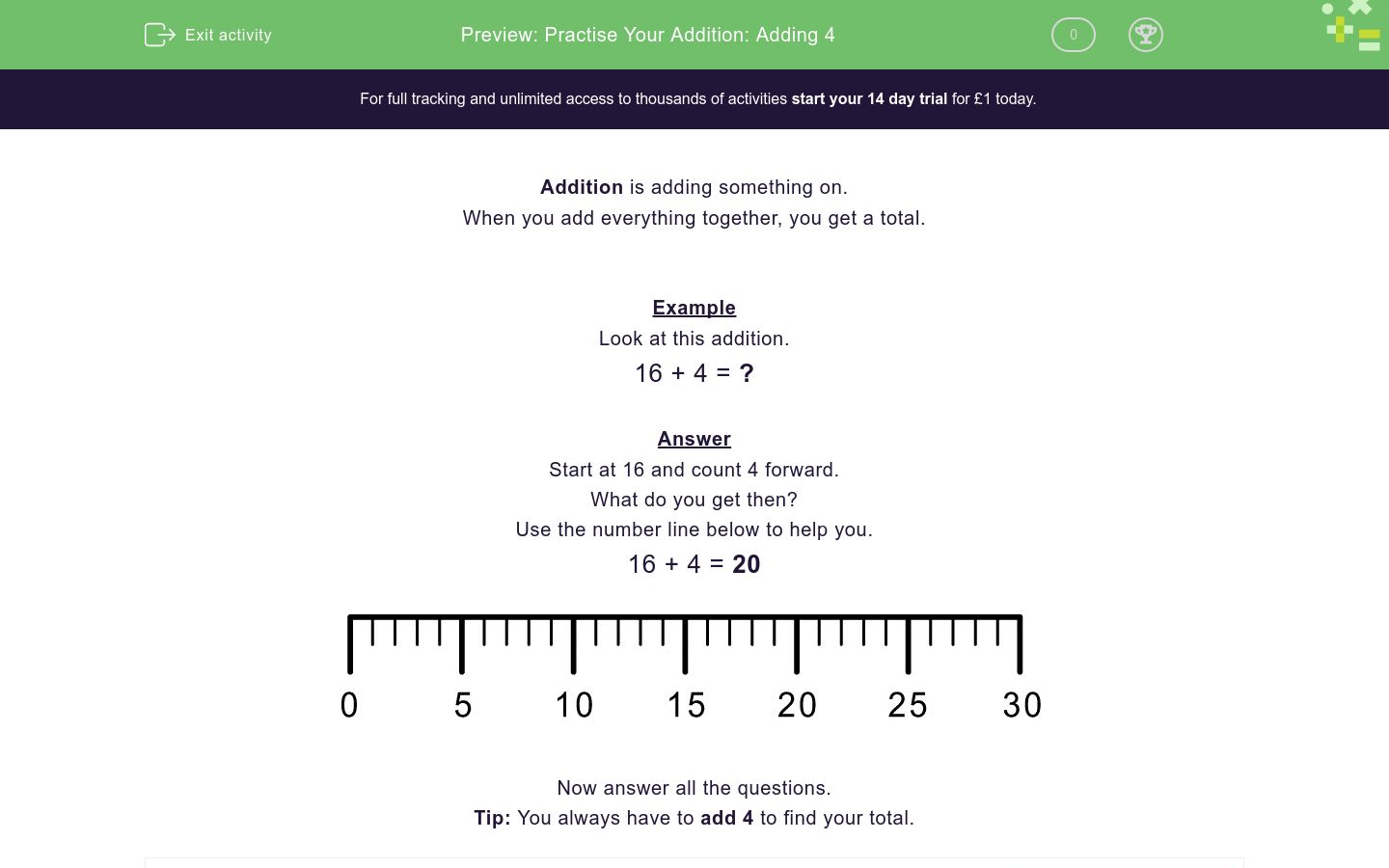### Affordable pricing from £10/month

In this worksheet, students practise adding 4 to numbers in the range 0-20.Key stage:  KS 1

Curriculum topic:   Number: Addition and Subtraction

Curriculum subtopic:   Add and Subtract to 20

Difficulty level:### QUESTION 1 of 10

When you add everything together, you get a total.

Example

16 + 4 = ?

Start at 16 and count 4 forward.

What do you get then?

16 + 4 = 20Write the missing number in the box below:

7 + 4 = ?Write the missing number in the box below:

13 + 4 = ?Write the missing number in the box below:

5 + 4 = ?Write the missing number in the box below:

10 + 4 = ?Write the missing number in the box below:

12 + 4 = ?Write the missing number in the box below:

16 + 4 = ?Write the missing number in the box below:

9 + 4 = ?Write the missing number in the box below:

19 + 4 = ?Write the missing number in the box below:

6 + 4 = ?Write the missing number in the box below:

17 + 4 = ?• Question 1

Write the missing number in the box below:

7 + 4 = ?11
EDDIE SAYS

Method

7 + 4 = 11

Missing Number = 11

• Question 2

Write the missing number in the box below:

13 + 4 = ?17
EDDIE SAYS

Method

13 + 4 = 17

Missing Number = 17

• Question 3

Write the missing number in the box below:

5 + 4 = ?9
EDDIE SAYS

Method

5 + 4 = 9

Missing Number = 9

• Question 4

Write the missing number in the box below:

10 + 4 = ?14
EDDIE SAYS

Method

10 + 4 = 14

Missing Number = 14

• Question 5

Write the missing number in the box below:

12 + 4 = ?16
EDDIE SAYS

Method

12 + 4 = 16

Missing Number = 16

• Question 6

Write the missing number in the box below:

16 + 4 = ?20
EDDIE SAYS

Method

16 + 4 = 20

Missing Number = 20

• Question 7

Write the missing number in the box below:

9 + 4 = ?13
EDDIE SAYS

Method

9 + 4 = 13

Missing Number = 13

• Question 8

Write the missing number in the box below:

19 + 4 = ?23
EDDIE SAYS

Method

19 + 4 = 23

Missing Number = 23

• Question 9

Write the missing number in the box below:

6 + 4 = ?10
EDDIE SAYS

Method

6 + 4 = 10

Missing Number = 10

• Question 10

Write the missing number in the box below:

17 + 4 = ?21
EDDIE SAYS

Method

17 + 4 = 21

Missing Number = 21

---- OR ----

Sign up for a £1 trial so you can track and measure your child's progress on this activity.

### What is EdPlace?

We're your National Curriculum aligned online education content provider helping each child succeed in English, maths and science from year 1 to GCSE. With an EdPlace account you’ll be able to track and measure progress, helping each child achieve their best. We build confidence and attainment by personalising each child’s learning at a level that suits them.

Get started